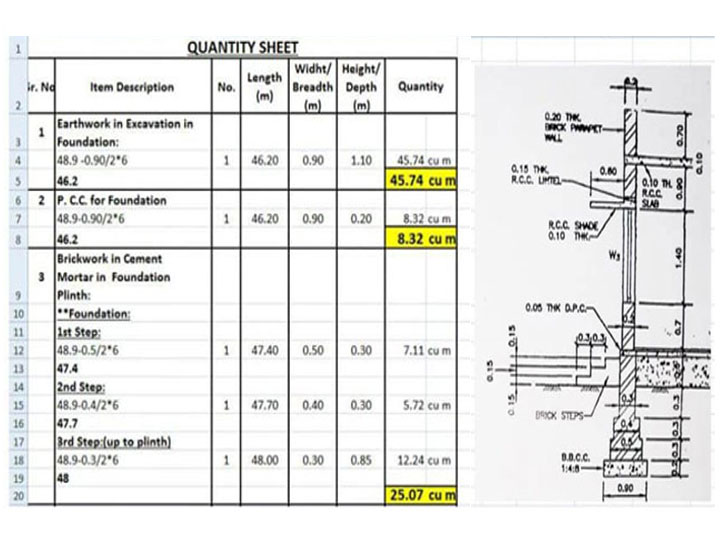Home Editor's Desk - Editorials Materials Quantity Estimation by Center Line Method

# Materials Quantity Estimation by Center Line Method

Quantity estimation is the process of finding out how many materials you need to build a structure, and how much will it cost you. Estimating the quantity of materials about a building project gives you a precise idea about how expensive the project is going to be and how much time will it take, which in turn lets you know if the project is feasible or not. Today, we will talk about the center line method of material quantity estimation.

The center line method is quite popular with small-scale builders because it is easy, quick and quite accurate. The only downside to this method is that it is only applicable for load-bearing buildings (where there are no columns or similar structural members). Hence it is mostly applied to only load-bearing walls, or partially in case of rooms with no columns.

Using this method and some formula, you can quickly find out the number of bricks and the amount of mortar and plaster required for the applicable room.

## Mechanisms of the center line method

The center line method of materials quantity estimation is one of the simplest of them available, and so it is very popular as well. If there are columns or other similar structures in the room, then this method cannot be used, but otherwise it’s a very good quantity estimation method.

The centerline method works on the principle that given all walls (long or short sides) have the same cross section and the same type of footing, the total volume of the wall will be the total length of the center line of all the walls in the room, multiplied by the cross section of the wall (height and breadth).

Hence, in this method the total center line length of the walls is measured out, with special care at the corners and junctions. When you have the total length of the walls, that is the center line length, you multiply it by the width and breadth of the wall – and that is the entire wall quantity. Now you can find out the materials estimate by using expected formulas or charts.

## Advantages of using the center line method

1. It is great for rooms with unusual types of walls, like circular rooms or polygonal shaped rooms, where other methods of quantity estimation will be very difficult indeed.
2. It is a very fast method for quantity estimation if the building has no cross walls.
3. It is a fast-paced method for quantity estimation.
4. It can be performed by field people and doesn’t require much expertise.

## Sample quantity estimation by center line method

We have, Total center line length
= 2 x (Length of long wall + Length of short wall) + 3 x (Length of partition wall)
So, Total center line length
= 2 x (5.3 + 5.3) + 3 x 4.3
= 34.1 m.
Earthwork in excavation
= Total center line length x Breadth x Depth
= 34.1 x 0.9 x (3 x 0.3)
= 34.1 x 0.9 x 0.9
= 27.621 m3.
Concrete in foundation
= Total center line length x Breadth x Depth.
= 34.1 x 0.9 x 0.3
= 9.207 m3.
Brickwork in foundation for first footing
= Total center line length x Breadth x Depth
= 34.1 x 0.6 x 0.3
= 6.138 m3.
Brickwork in foundation for second footing
= Total center line length x Breadth x Depth
= 34.1 x 0.5 x 0.3
= 5.115 m3.
Brickwork in plinth
= Total center line length x Breadth x Depth
= 34.1 x 0.3 x 0.6
= 6.138 m3.
Brickwork in superstructure
= Total center line length x Breadth x Depth
= 34.1 x 0.3 x 3.5
= 35.805 m3.

## Important considerations in the center line method

• In case of buildings with one partition wall or cross wall having two junctions, for earthwork in foundation trench and foundation concrete, deduction of one breadth of trench or concrete from total center length.
• o For each junction half breadth of the respective item is to be deducted from the total center length.
• For footings, deduction of one breadth from the total center length is to be done for two junctions.
• In case of number of footings, the length of first footing is determined by applying half breadth as deduction per junction from the total center line length
• o For each subsequent footing, simply offset for every junction to the length of previous footing.
• At every stage deduction of half breadth of the main wall at that particular level shall have to be made per junction. That means the overall one breadth for two junctions from the total center line length.
• o This net center length after deduction shall be multiplied by the respective breadth and depth to get total quantities.
• If two walls come from opposite directions and they meet at the same point, there will be two junctions.
• For buildings having different type of walls, each set of walls have to be considered separately.
• o All the outer walls or main walls shall be considered first together and then all inter or cross walls must be considered.
• There is no provision of deduction for main walls or outer walls. However, deduction of half breadth of main wall, for each junction must be made for inter or cross walls.